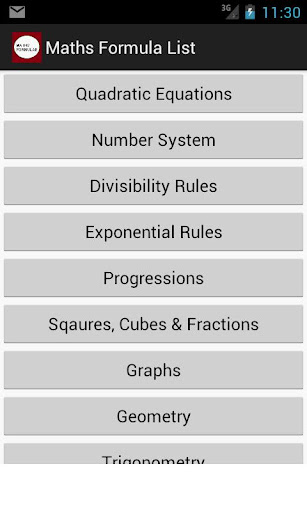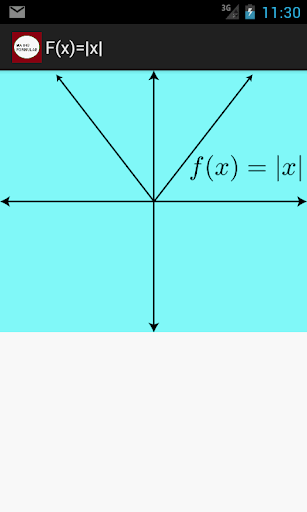Maths Formula ListThese are very useful maths formulas that serve as a quick reference.

This includes topics:

Algebra:

List of Squares, Cubes, Fourths & fifths

List of Percentages, Fractions, Decimals

Logarithms

Exponents

Trigonometry

Geometry

Area and Perimeter of Circle, Square, Rectangles, Parallelogram, Ring, Sector, Trapezoid, Equilateral TriangleVolume and Surface Area of Cone, Cube, Cylinder, Frustum, Pyramid, Sphere

Triangle Properties, Quadrilateral Properties, Pentagon Properties

Coordinate Geometry

Line Equation, Line Slope, Line Midpoint, Slope of Parallel Lines, Slope of Perpendicular Lines

Circle Equation

Binomial Theorem

Arithmetic Progression, Geometric Progression & Harmonic Progression

Divisibility Rules

Probability

and a lot more...

This Formulas Collection is useful for MBA Exams such as Common Aptitude Test (CAT - IIM), Management Aptitude Test (MAT), Xavier Admission Test (XAT) and other MBA exams (JMET,ATMA,SNAP,NMAT,IIFT,FMS)

This will also be useful for GRE, GMAT,SAT, IIT JEE, AIEEE, etc.

It also has Maths formulas for College Grade/Higher Grade Students/School Students.

It lists out all the important formulas/topics in Algebra, Geometry, Trigonometry. Regular review of these formulas/concepts will definitely help improve your grades.

Helps in all the following ways:

Maths, Mathematics, Maths formulas, Mathematics formulas, Maths formulae, Mathematics formulae